# Vacuum heating calculation

• ektov_konstantin
In summary, the conversation discusses the calculation of heating time for polycor substrates in a vacuum chamber using a simple mathematical model. The goal is to determine the time when conductive heating equals radiative heat losses. However, the results obtained from the calculation are not physically realistic, and there may be issues with units. The conversation also mentions conducting a heat transfer calculation in ANSYS to consider all radiation heating and conductive losses. f

#### ektov_konstantin

TL;DR Summary
I have a physically correct approach but I get incorrect result and need some help!
Hello, everyone!

I am calculating the time of substrates' heating in the vacuum chamber.

It is a simple mathematical model to calculate roughly how much time does it take to heat polycor substrates in the vacuum chamber.

At least all the tube heaters power heats the substrates (this is because the geometry of the vacuum chamber and beacuse all inner surface is polished).

So our goal is to get the time when conductive heating will be equal to the radiative heat losses.

Look at the scheme.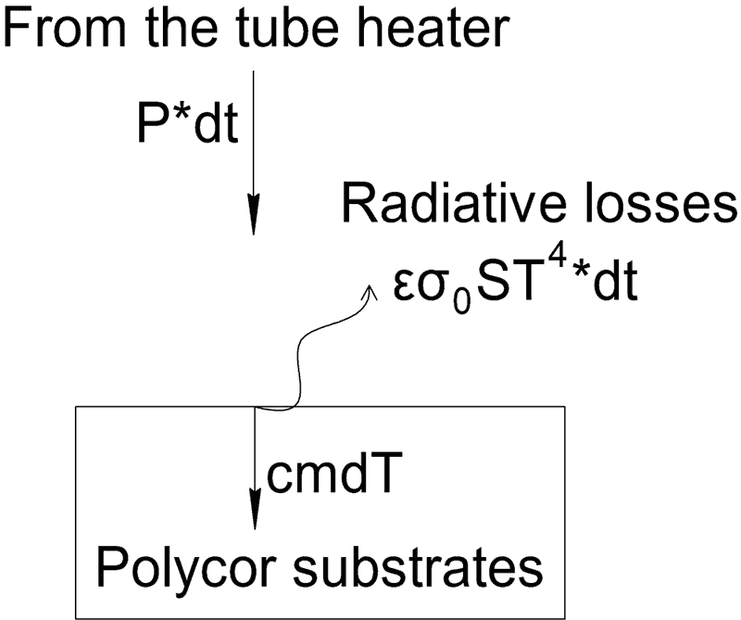So the differential equation is .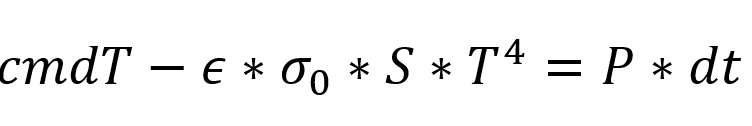And dividing the variables we get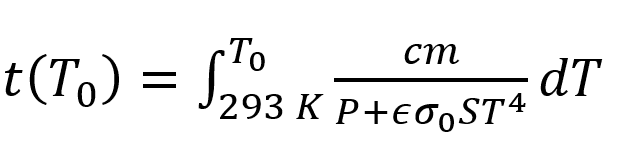, where T_0 is the Temperature when conductive heating equals radiative losses.

If P=800 W, I have this dependence...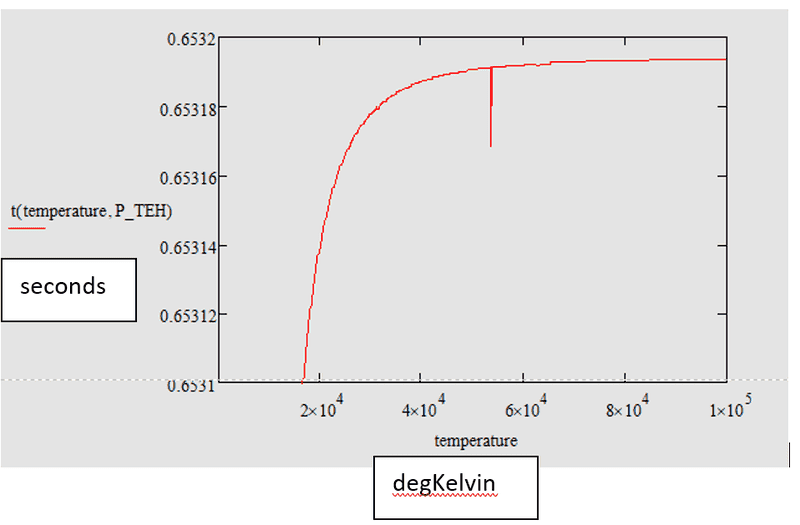and as you can see, it is not physically right beacuse of two reasons. First - why there is a horisontal asymptote (it must be vertical!) ? Second - the heating is very-very fast...

For instance, I have calculated the heating time if there are no radiative losses.

t = c*m*(T_0-293)/W

and for W=800 W, T_0=623 K (technological process temperature) we get t=0,205 seconds - and it is rubbish too I think...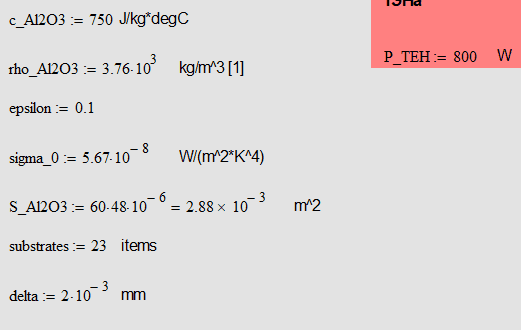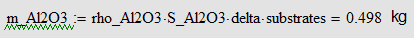1. Is ALL of the thermal energy of the heater focused on the work piece?
• How?
2. What about conductive losses from the heater thru its mounting?
3. Is the work piece suspended and not in contact with a support that gives a path for conductive losses?
• How?

The graph shows a temperature of 1×105K (100 000 Kelvin); is that realistic? (Have you obtained a Star for a heat source?)

Graphs generally have the independent variable (Time) on the X-axis, and the dependent variable (Temperature) on the Y-axis; that makes them easier for most of us to interpret.

Anxiously awaiting clarifications of the above!
Tom

•Lnewqban
1. Is ALL of the thermal energy of the heater focused on the work piece?
• How?
2. What about conductive losses from the heater thru its mounting?
3. Is the work piece suspended and not in contact with a support that gives a path for conductive losses?
• How?

The graph shows a temperature of 1×105K (100 000 Kelvin); is that realistic? (Have you obtained a Star for a heat source?)

Graphs generally have the independent variable (Time) on the X-axis, and the dependent variable (Temperature) on the Y-axis; that makes them easier for most of us to interpret.

Anxiously awaiting clarifications of the above!
Tom
Hello, Tom!

Imagine, that this is abstract mathematical model.
I extremely agree with you that...
1. There are conductive losses in the heater mounting and to the polycor substrates basement (this is round plate)
2. Not all the power from the heater heats the substrates but if I have to make this assumption to ease my calculation. It is affordable because all inner surface of the chamber is polished.

Later I will make the heat transfer calculation in ANSYS application. It will consider all radiation heating and conductive losses.

BUT my mathematical calculation gives unphysical and illogical results.

Just imagine that this is an abstract body... And losses are radiative. Temperature must come to stationary value. But it doesn't. What I get is that time (!) becomes static. Time stops. The question is why?!

My differential equation is solved correct - I have rechecked it.

Your equation seems to be correct.
You are basically looking for the time that it takes to reach thermal equilibrium between the heater and the polycor within the vacuum chamber.

https://www.engineeringtoolbox.com/heat-up-energy-d_1055.html

The calculations may have unit's problems if the result is not logical.

Your diagram should look something like this: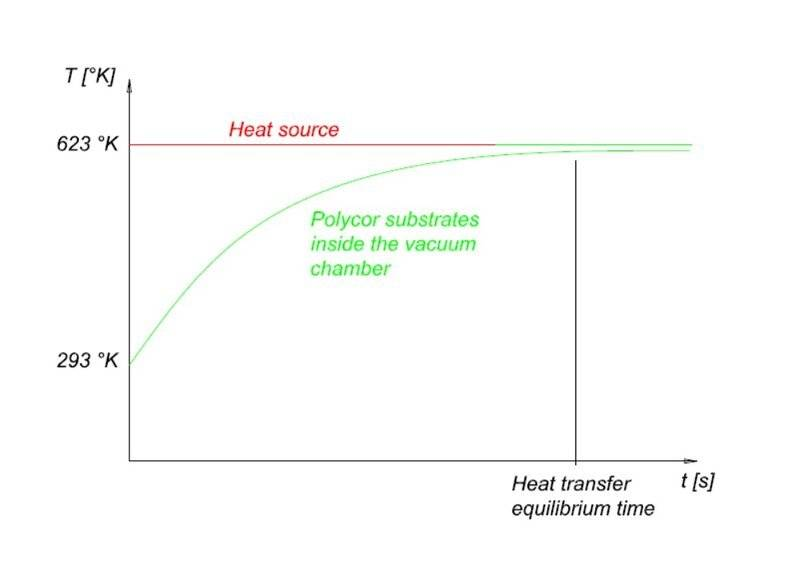Your equation seems to be correct.
The calculations may have unit's problems if the result is not logical.
Your diagram should look something like this:

View attachment 297387
Yes. Thats right. Thank you for your answer. But Ive checked units. They are all in SI... May I show you my MathCAD file?

Yes. Thats right. Thank you for your answer. But Ive checked units. They are all in SI... May I show you my MathCAD file?
You are welcome.